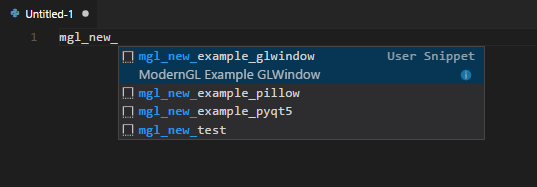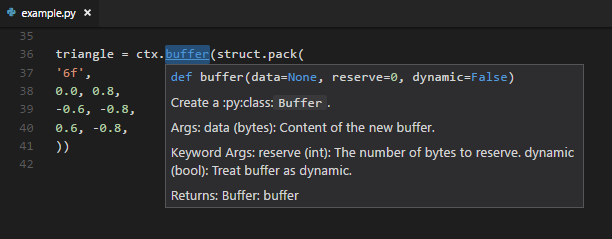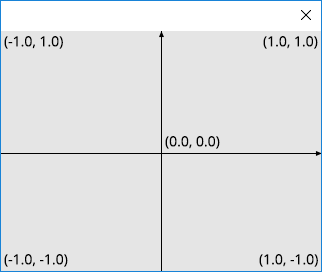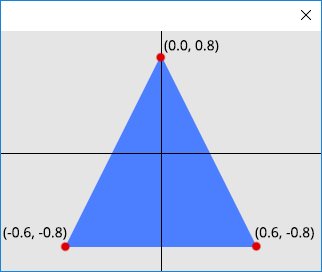# TL;DR¶

For those who want to learn from examples.

## Install¶

```pip install ModernGL
pip install Pillow numpy
```

## Choose a Window¶

```pip install PyQt5
pip install GLWindow
pip install pygame
pip install pyglet
pip install PyOpenGL
```

## Snippets¶

2. Open vscode
3. Press `ctrl+shift+P`
4. Select `Open User Snippets`
5. Select `Python`
6. Paste some snippets from vscode snippets

Warning

Do not replace the python.json if you have python snippets already.

1. Create a new python file
2. Type `mgl_new_` (not working? -> see the note below)Note

• Press `ctrl+space` to trigger suggestions.
• Try to save an empty file with `.py` ending.
• Select the language in the right left corner of the window.
• Make sure you have pasted the snipptes correctly.
• Press `ctrl+shift+P` then select `Insert Snippet` then type `mgl_new_`

All the ModernGL snippets starts with `mgl_`

## Linting¶

Full linting support is provided:## Hello World with PyQt5¶

```import struct

import ModernGL
from PyQt5 import QtOpenGL, QtWidgets

class QGLControllerWidget(QtOpenGL.QGLWidget):
def __init__(self):
fmt = QtOpenGL.QGLFormat()
fmt.setVersion(3, 3)
fmt.setProfile(QtOpenGL.QGLFormat.CoreProfile)
fmt.setSampleBuffers(True)
super(QGLControllerWidget, self).__init__(fmt, None)

def initializeGL(self):
self.ctx = ModernGL.create_context()

prog = self.ctx.program([
#version 330
in vec2 vert;
void main() {
gl_Position = vec4(vert, 0.0, 1.0);
}
'''),
#version 330
out vec4 color;
void main() {
color = vec4(0.3, 0.5, 1.0, 1.0);
}
'''),
])

vbo = self.ctx.buffer(struct.pack('6f', 0.0, 0.8, -0.6, -0.8, 0.6, -0.8))
self.vao = self.ctx.simple_vertex_array(prog, vbo, ['vert'])

def paintGL(self):
self.ctx.viewport = (0, 0, self.width(), self.height())
self.ctx.clear(0.9, 0.9, 0.9)
self.vao.render()
self.ctx.finish()

app = QtWidgets.QApplication([])
window = QGLControllerWidget()
window.show()
app.exec_()
```

## Hello World with GLWindow¶

```import struct

import GLWindow
import ModernGL

wnd = GLWindow.create_window()
ctx = ModernGL.create_context()

prog = ctx.program([
#version 330
in vec2 vert;
void main() {
gl_Position = vec4(vert, 0.0, 1.0);
}
'''),
#version 330
out vec4 color;
void main() {
color = vec4(0.3, 0.5, 1.0, 1.0);
}
'''),
])

vbo = ctx.buffer(struct.pack('6f', 0.0, 0.8, -0.6, -0.8, 0.6, -0.8))
vao = ctx.simple_vertex_array(prog, vbo, ['vert'])

while wnd.update():
ctx.viewport = wnd.viewport
ctx.clear(0.9, 0.9, 0.9)
vao.render()
```

## Rendering¶

To render we will need:

1. `Context` — OpenGL support
2. `Program` — Shaders
3. `Buffer` filled with the vertex data.
4. `VertexArray` to connect the buffer and the program.

Note

The ModernGL implementation of the `VertexArray` stores a reference to the program, the original OpenGL implementation for Vertex Arrays does not provide this.

To see what other things are different please see: ModernGL vs OpenGL

### Context¶

The context can be created once the window is ready.

```ctx = ModernGL.create_context()
```

The `ctx` provides functionality to create the `Program`, `Buffer` and `VertexArray`

### Program¶

A Program Object represents fully processed executable code, in the OpenGL Shading Language.

We will create a simple program from a vertex and fragment shader.

Takes the vertices as the input.

```#version 330
in vec2 vert;
void main() {
gl_Position = vec4(vert, 0.0, 1.0);
}
```

Writes the output to the screen.

```#version 330
out vec4 color;
void main() {
color = vec4(0.3, 0.5, 1.0, 1.0);
}
```

The entire program can be created:

```prog = ctx.program([
#version 330
in vec2 vert;
void main() {
gl_Position = vec4(vert, 0.0, 1.0);
}
'''),
#version 330
out vec4 color;
void main() {
color = vec4(0.3, 0.5, 1.0, 1.0);
}
'''),
])
```

### Buffer¶

The buffer in the example is created with the following line of code:

```vbo = ctx.buffer(struct.pack('6f', 0.0, 0.8, -0.6, -0.8, 0.6, -0.8))
```

The triangle is given as:

```#   x     y
0.0,  0.8
-0.6, -0.8
0.6, -0.8
```The window coordinatesThe triangle in window coordinates

### VertexArray¶

We want the following to happen:

1. Feed the vertex shader with the coordinates of the triangle.
2. The vertex shader transforms the triangle to screen coordinates. (we gave the vertices in window coordinates so the vertex shader just passes the coordinates towards)
3. The triangle will be rasterized.
4. The triangle gets “painted” with the color given in the fragment shader.

In the example the VertexArray is created with the following line of code:

```vao = ctx.simple_vertex_array(prog, vbo, ['vert'])
```

The `simple_vertex_array()` creates a `VertexArray` object. The VertexArray will ensure that the `prog` program object will be used when rendering. Additionally detects the format of the `in vec2 vert` and binds the attribute to the buffer.

Then finally the rendering is done by:

```vao.render()
```

The expression above expands to:

```vao.render(ModernGL.TRIANGLES, vertices=3)
```

Note

The `render()` method’s first parameter is the rendering mode (by default `ModernGL.TRIANGLES` are rendered). The numer of vertices to render is detected form the size of the buffer assigned to the `vert` vertex attribute. The `vbo` has `6f` (six floats), with the total of 24 bytes, The `vert` vertex attribute is a `vec2` with the size of 8 bytes. The number of vertices detected is `24 / 8 = 3`. It can be read/changed programatically by using the `VertexArray.vertices` attibute.

Note

To create a complex VertexArray use the `Context.vertex_array()` method.

• Multiple buffers can be used to create VertexArrays.
• Padding, Per Vertex, Per Instance and Per Render attributes are also supported.
• Individual vertex attributes can be bound to different buffers with a custom offset, stride and divisor specified.